# RD Sharma Solutions -Ex-19.2, (Part -3), Surface Area And Volume Of Right Circular Cylinder, Class 9 Notes | Study RD Sharma Solutions for Class 9 Mathematics - Class 9

## Class 9: RD Sharma Solutions -Ex-19.2, (Part -3), Surface Area And Volume Of Right Circular Cylinder, Class 9 Notes | Study RD Sharma Solutions for Class 9 Mathematics - Class 9

The document RD Sharma Solutions -Ex-19.2, (Part -3), Surface Area And Volume Of Right Circular Cylinder, Class 9 Notes | Study RD Sharma Solutions for Class 9 Mathematics - Class 9 is a part of the Class 9 Course RD Sharma Solutions for Class 9 Mathematics.
All you need of Class 9 at this link: Class 9

Q.23 A cylindrical container with diameter of base 56 cm contains sufficient water to submerge a rectangular solid of iron with dimensions 32 cm x 22 cm x 14 cm . Find the rise in the level of the water when the solid is completely submerged.

Solution:

Given data is as follows :

Diameter of cylinder = 56 cm

Dimensions of rectangular block = 32 cm × 22 cm × 14 cm

We have to find the raise in the level of water in the cylinder  .

First let us find the raise in the level of water in the cylinder . Diameter is given as 56 cm . Therefore ,

r = 28 cm

We know that the raise in the volume of water displaced in the cylinder will be equal to the volume of the rectangular block .

Let the raise in the level of water be h . Then we have ,

Volume of cylinder of height h and radius 28 cm = Volume of the rectangular block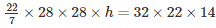h = 4 cm

Therefore , the raise in the level of water when the rectangular block is immersed in the cylinder is 4 cm.

Q. 24. A cylindrical tube , open at both ends , is made of metal . The internal diameter of the tube is 10.4 cm and its length is 25 cm . The thickness of the metal is 8 mm everywhere . Calculate the volume of the metal .

Solution:

Given data is as follows :

Internal diameter = 10.4 cm

Thickness of the metal = 8 mm

Length of the pipe = 25 cm

We have to find the volume of the metal used in the pipe .

We know that ,

Volume of the hollow pipe = π(R2−r2)h

Given is the internal diameter which is equal to 10.4 cm .Therefore ,

r = 10.4/2

r = 5.2 cm

Also , thickness is given as 8 mm . Let us convert it to centimeters .

Thickness = 0.8 cm

Now that we know the internal radius and the thickness of the pipe , we can easily find external radius ‘R’ .

R = 5.2 + 0.8

R = 6 cm

Therefore , Volume of metal in the pipe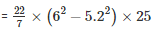= 704 cm3

Therefore , the volume of metal present in the hollow pipe is 704 cm3 .

Q. 25 . From a tap of inner radius 0.75 cm , water flows at the rate of 7 m per second . Find the volume in litres of water delivered by the pipe in one hour .

Solution:

Given data is as follows :

r = 0.75 cm

Water flow rate = 7 m/sec

Time = 1 hour

We have to find the volume of water that flows through the pipe for 1 hour .

Let us first convert water flow from m/sec to cm/sec , since radius of the pipe is in centimeters

We have  ,

Water  flow rate =  7 m/sec

= 700 cm/sec

Volume of water delivered by the pipe is equal to the volume of a cylinder with h = 7 m and r = 0.75 cm . Therefore ,

Volume of water delivered in 1 second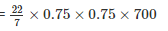We have to find the volume of water delivered in 1hour which is nothing but 3600 seconds.

Therefore , we have

Volume of water delivered in 3600 seconds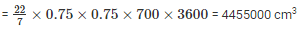We know that 1000 cm= 1 litre

Therefore ,

Volume of water delivered in 1 hour = 4455 liters

Therefore , Volume of water delivered by the pipe in 1 hour is equal to 4455 liters.

Q. 26. A cylindrical water tank of diameter 1.4 m and height 2.1 m is being fed by a pipe of diameter 3.5 cm through which water flows at the rate of 2 metre per second . In how much time the tank will be filled ?

Solution:

Given data is as follows  :

Diameter of the tank = 1.4 m

Height of the tank = 2.1 m

Diameter of the pipe = 3.5 cm

Water flow rate = 2 m/sec

We have to find the time required to fill the tank using the pipe .

The diameter of the tank is given which is 1.4 m . Let us find the radius .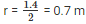Volume of the tank = πr2h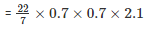Given is the diameter of the pipe which is 3.5 cm . Therefore , radius is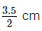Let us convert it into metres . It then becomes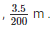Volume of water that flows through the pipe in 1 second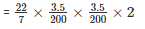Let the time taken to fill the tank be x seconds . Then we have ,

Volume of water that flows through the pipe in x seconds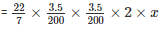We know that volume of the water that flows through the pipe in x seconds will be equal to the volume of the tank . Therefore , we have

Volume of water that flows through the pipe in x seconds  = Volume of the tank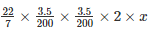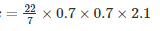x = 1680 seconds

x = 1680/60 minutes

x = 28 minutes

Hence , it takes 28 minutes to fill the tank using the given pipe .

Q.27. A rectangular sheet of paper 30 cm x 18 cm can be transformed into the curved surface of a right circular cylinder in two ways i.e. , either by rolling the paper along its length or by rolling it along its breadth . Find the ratio of the volumes of the two cylinders thus formed .

Solution:

Given data is as follows :

Dimensions of the rectangular sheet of paper = 30 cm × 18 cm

We have to find the ratio of the volumes of the cylinders formed by rolling the sheet along its length and along its breadth .

Let V1 be the volume of the cylinder which is formed by rolling the sheet along its length .

When the sheet is rolled along its length , the length of the sheet forms the perimeter of the cylinder . Therefore , we have ,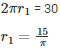The width of the sheet will be equal to the height of the cylinder . Therefore ,

h= 18 cm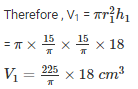Let V2 be the volume of the cylinder formed by rolling the sheet along its width .

When the sheet is rolled along its width , the width of the sheet forms the perimeter of the base of the cylinder . Therefore , we have ,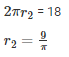The length  of the sheet will be equal to the height of the cylinder . Therefore ,

h= 30 cm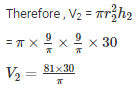Now that we have the volumes of two cylinders , we have ,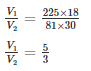Therefore , the ratio of the volumes of the two cylinders is 5 : 3 .

Q.28. How many litres of water flow out of a pipe having an area of cross-section of 5 cm2  in one minute ,  if the speed of water in the pipe is 30 cm/sec ?

Solution:

Given data is as follows :

Area of cross section of the pipe = 5 cm2

Speed of water = 30 cm/sec

We have to find the volume of water that flows through the pipe in 1 minute .

Volume of water that flows through the pipe in one second = πr2h

Here , πr2 is nothing but the cross section of the pipe and h is 30 cm .

Therefore , we have ,

Volume of water that flows through the pipe in one second = 5×30=150cm3

Volume of water that flows through the pipe in one minute = 150×60=9000cm3

We know that 1000 cm3 = 1 litre . Therefore ,

Volume of water that flows through the pipe in one minute = 9 litres

Hence , the volume of water  that flows through the given pipe in 1 minute is 9 litres .

Q.29. The sum of the radius of the base and height of a solid cylinder is 37 m. If the total surface area of the solid cylinder is 1628 cm2 . Find the volume of the cylinder .

Solution:

Given data is as follows :

h + r = 37 cm

Total surface area of the cylinder = 1628 cm2

That is ,

2πrh+2πr2 = 1628

2πr(h+2r) = 1628

But it is already given in the problem that ,

h + r = 37 cm

Therefore , 2πr×37 = 1628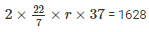r = 7 cm

Since , h + r = 37 cm

We have , h + 7 = 37 cm

H = 30 cm

Now that we know both height and radius of the cylinder , we can easily find the volume .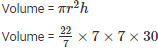Volume = 4620 cm 3

Hence , the volume of the given cylinder is 4620 cm3 .

Q.30. Find the cost of sinking a tube well 280 m deep, having diameter 3 m at the rate of Rs 3.60 per cubic metre. Find also the cost of cementing its inner curved surface at Rs 2.50 per square metre .

Solution:

Given data is as follows :

Height of the tube well = 280 m

Diameter = 3 m

Rate of sinking of the tube well = Rs. 3.60/m3

Rate of cementing = Rs. 2.50/m2

Given is the diameter of the tub well which is 3 metres . Therefore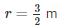Volume of the tube well = πr2h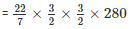= 1980 m2

Cost of sinking the tube well = Volume of the tube well × Rate of sinking the tube well  = 1980 × 3.60

= Rs . 7128

Curved surface area = 2πrh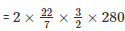= 2640 m2

Cost of cementing = Curved Surface area × Rate of cementing

= 2640 × 2.50

= Rs . 6600

Therefore , the total cost of sinking the tube well is Rs . 7128 and the total cost of cementing  its inner surface is Rs . 6600 .

Q.31. Find the length of 13.2 kg of copper wire of diameter 4 mm, when 1 cubic cm of copper weighs 8.4 gm .

Solution:

Given data is as follows :

Weight of copper  wire = 13.2 kg

Diameter = 4 mm

Density = 8.4 gm /cm3

We have to find the length of the copper wire .

Given is the diameter of the wire which is 4 mm . Therefore ,

r = 2 mm

Let us convert r from millimeter to centimeter , since density is in terms of gm/cm3 . Therefore ,

r = 2/10 cm

Also , weight of the copper wire is given in kilograms . Let us convert into grams since density is in terms of gm/cm3 . Therefore , we have ,

Weight of copper wire = 13.2 × 1000 gm

= 13200 gm

We know that

Volume × Density = Weight

Therefore , πr2h×8.4 = 13.2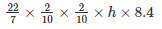h = 12500 cm

h = 125 m

Hence , the length of the copper wire is 125 metres .

Q.32. A well with 10 m inside diameter is dug 8.4 m deep. Earth taken out of it is spread all around it to a width of 7.5 m to form an embankment. Find the height of the embankment.

Solution:

Given  data is as follows :

Inner diameter of the well = 10 m

Height = 8.4 m

Width of embankment = 7.5 m

We have to find the height of the embankment.

Given is the diameter of the well which is 10 m. Therefore ,

r = 5 m

The outer radius of the embankment,

R = Inner radius of the well + width of the embankment

= 5 + 7.5

= 12.5 m

Let H be the height of the embankment.

The volume of earth dug out is equal to the volume of the embankment . Therefore ,

Volume of embankment = Volume of earth dug out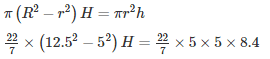H = 1.6 m

Thus , height of the embankment is 1.6 m.

The document RD Sharma Solutions -Ex-19.2, (Part -3), Surface Area And Volume Of Right Circular Cylinder, Class 9 Notes | Study RD Sharma Solutions for Class 9 Mathematics - Class 9 is a part of the Class 9 Course RD Sharma Solutions for Class 9 Mathematics.
All you need of Class 9 at this link: Class 9Use Code STAYHOME200 and get INR 200 additional OFF

## RD Sharma Solutions for Class 9 Mathematics

91 docs

Track your progress, build streaks, highlight & save important lessons and more!

,

,

,

,

,

,

,

,

,

,

,

,

,

,

,

,

,

,

,

,

,

,

,

,

,

,

,

,

,

,

;